Next: Bibliography Up: On Regularity for Weak Previous: On Regularity for Weak

# Introduction

We consider the Cauchy problem for the porous media equation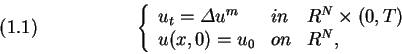where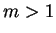and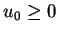. By a solution of (1.1) we mean a function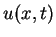such that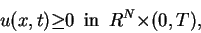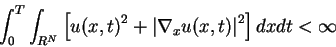and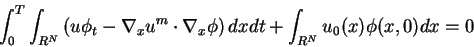for any continuously differentiable function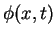with compact support in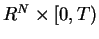. The problem(1.1) has been studied by many authors. For a detailed account of (1.1) we refer to the survey of Kalashnikov . The existence and the uniqueness of solutions of (1.1) are due to  and  under some assumption on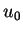.

We are concerned to the regularity property for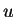. The local Hölder continuity ofwas shown by Caffarelli and Friedman . Aronson and Bénilan  proved that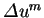belongs to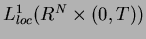. The method of their proof is to obtain the inequarity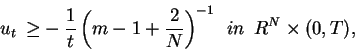which is in the distribution sence. Soon after Bénilan  proved that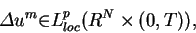if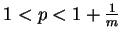. Here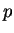needs to be less than 2 in virtue of. When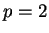, there is the following result by one of the authors :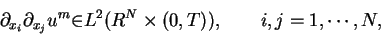if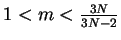. Whenis spherically symmetric under some assumption on, this result was improved in  as follows: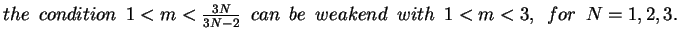We consider the well-known Barenblatt solution: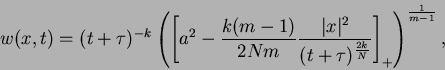where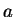,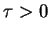and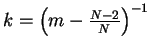. We rewrite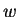simply with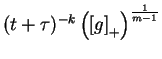. Then obviously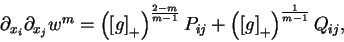where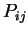and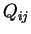are smooth functions. Hence we see that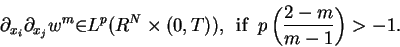Letbe the spherically symmetric solution of (1.1). Then from the above we conjecture that for any given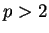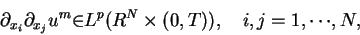if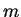is close to 1. In this paper our first aim is to verify this conjecture (see Theorem 1 ). Secondly we give precisely the admissive value ofin Theorem 1, when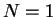(see Theorem 2). The tool is to prepare some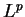- estimates with a weight by the integreation by parts.Next: Bibliography Up: On Regularity for Weak Previous: On Regularity for Weak
Nobuki Takayama 2002-04-24